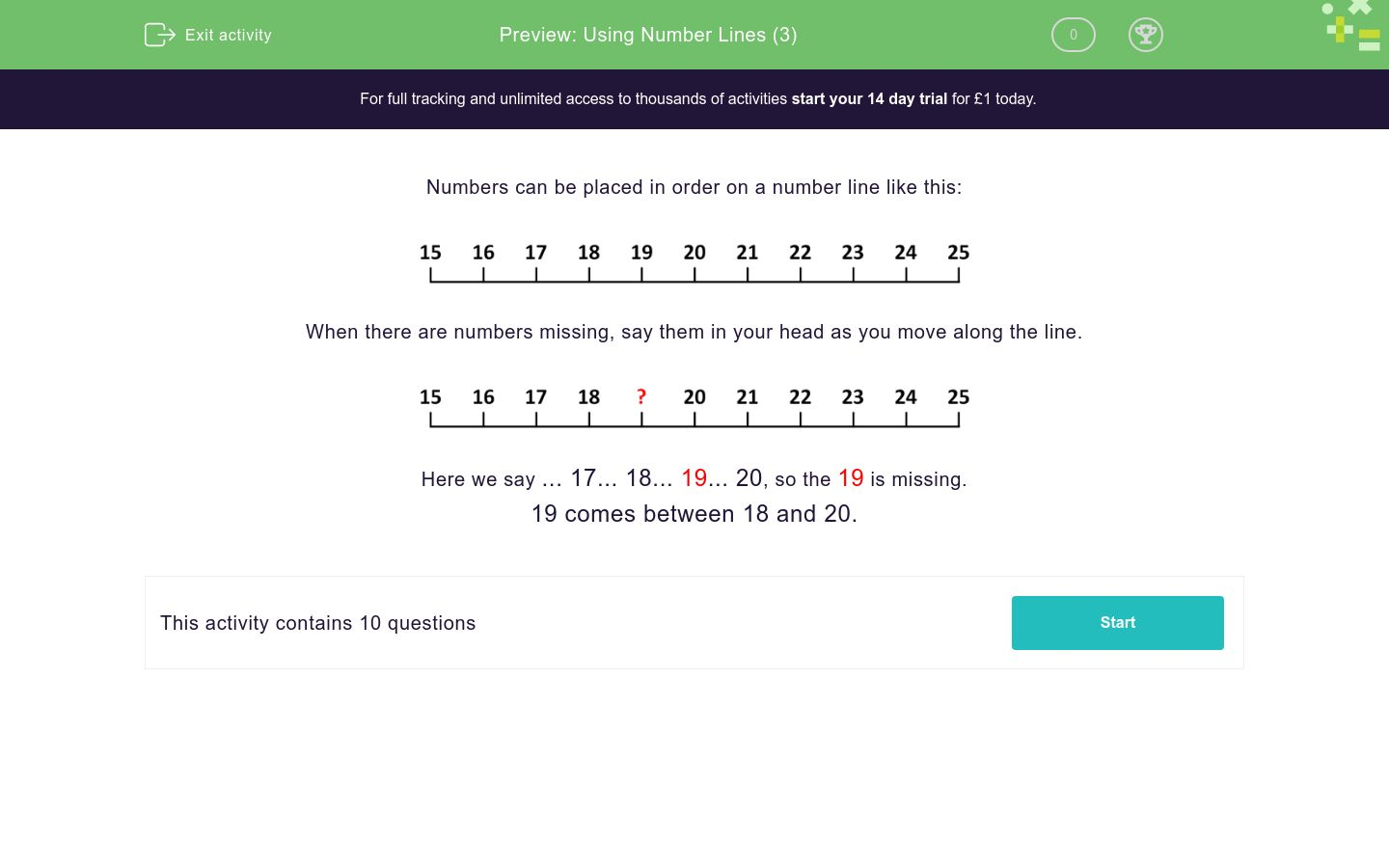# Using Number Lines (3)

In this worksheet, students must identify the missing number on a number line.Key stage:  KS 1

Curriculum topic:   Number: Number and Place Value

Curriculum subtopic:   Represent Numbers to 100

Difficulty level:### QUESTION 1 of 10

Numbers can be placed in order on a number line like this:When there are numbers missing, say them in your head as you move along the line.Here we say ... 17... 18... 19... 20, so the 19 is missing.

19 comes between 18 and 20.

Write the number which goes where the ? is found on this number line.Write the number which goes where the ? is found on this number line.Write the number which goes where the ? is found on this number line.Write the number which goes where the ? is found on this number line.Write the number which goes where the ? is found on this number line.Write the number which goes where the ? is found on this number line.Write the number which goes where the ? is found on this number line.Write the number which goes where the ? is found on this number line.Write the number which goes where the ? is found on this number line.Write the number which goes where the ? is found on this number line.• Question 1

Write the number which goes where the ? is found on this number line.17
EDDIE SAYS
17 comes between 16 and 18.
• Question 2

Write the number which goes where the ? is found on this number line.21
EDDIE SAYS
21 comes between 20 and 22.
• Question 3

Write the number which goes where the ? is found on this number line.25
EDDIE SAYS
25 comes after 24.
• Question 4

Write the number which goes where the ? is found on this number line.31
EDDIE SAYS
31 comes between 30 and 32.
• Question 5

Write the number which goes where the ? is found on this number line.36
EDDIE SAYS
36 comes between 35 and 37.
• Question 6

Write the number which goes where the ? is found on this number line.40
EDDIE SAYS
40 comes between 39 and 41.
• Question 7

Write the number which goes where the ? is found on this number line.21
EDDIE SAYS
21 comes before 22.
• Question 8

Write the number which goes where the ? is found on this number line.55
EDDIE SAYS
55 comes between 54 and 56.
• Question 9

Write the number which goes where the ? is found on this number line.60
EDDIE SAYS
60 comes between 59 and 61.
• Question 10

Write the number which goes where the ? is found on this number line.89
EDDIE SAYS
89 comes before 90.
---- OR ----

Sign up for a £1 trial so you can track and measure your child's progress on this activity.

### What is EdPlace?

We're your National Curriculum aligned online education content provider helping each child succeed in English, maths and science from year 1 to GCSE. With an EdPlace account you’ll be able to track and measure progress, helping each child achieve their best. We build confidence and attainment by personalising each child’s learning at a level that suits them.

Get started